## Layered Noise

So far the noise we generated always looked either very soft, or very noisy. We can improve that by layering multiple layers of noise on top of each other. That way we get the structure of the soft noise as well as the interresting details of the more high frequency noise. Layering noise works well for value noise as well as perlin noise. While layering noise might give you patterns that are closer to what you intend to see, you also have to be careful if you worry about performance because each layer of noise you add costs you about as much performance as the first.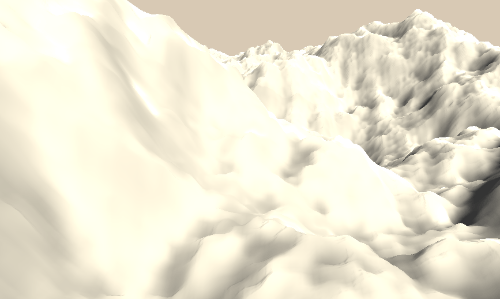## Layered 1d Noise

We can already change the frequency, and by that change the roughness of the noise. We do that by changing the noise size variable.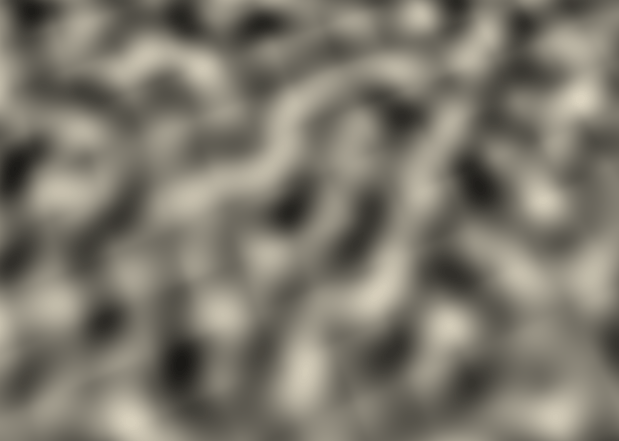As in the previous tutorials, we’ll start by implementing the new technique in 1d. We start by sampling the noise twice, once like we did it before and another time with the noise value multiplied by 2. Multiplying the value means that the noise will change faster and be more high-frequency. After sampling the noise twice we add the second, more high-frequent noise, with less strength to the fist noise. This is possible with perlin noise because it is around 0, so it adds and subtracts values, if you use value noise I recommend remapping it around 0 (by multiplying it by 2 and subtracting 1) first and then adding the noise values.

For ease of reading we’ll also put the code we use for sampling noise in it’s own function.

``````float sampleLayeredNoise(float value){
float highFreqNoise = gradientNoise(value * 6);
noise = noise + highFreqNoise * 0.2;
return noise;
}
``````
``````void surf (Input i, inout SurfaceOutputStandard o) {
float value = i.worldPos.x / _CellSize;
float noise = sampleLayeredNoise(value);

float dist = abs(noise - i.worldPos.y);
float pixelHeight = fwidth(i.worldPos.y);
float lineIntensity = smoothstep(2*pixelHeight, pixelHeight, dist);
o.Albedo = lerp(1, 0, lineIntensity);
}
``````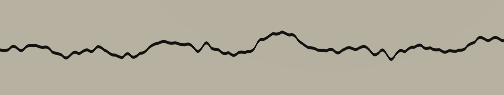This already gives us some of the effect we want, a overall structure with some rougher detail, but we can easily go further by sampling the noise even more often. The amount of times we sample the noise is also called octaves, so the example we have right now would be layered noise with 2 octaves.

To implement more octaves we will implement the sampling in a loop. We will also make the octaves less set in stone, instead we use a define statement to set it as a constant in our code. We could also make it completely variable, but then the compiler wouldn’t be able to optimize our loop by unrolling it so I’d like to avoid that. The frequency of each layer will be a multiple of the one before it, we will put that frequency multiplier in a variable and call it roughness. The amount which the layer will be factored in will be a fraction of the amount the previous layer is factored in, we’ll call that factor persistance. I also factored in the number of the loop into the noise sample, so that we don’t get weird artefacts at 0/0 where multiplying the value doesn’t change it which would lead to the same value being returned by the noise function for all layers.

``````Properties {
_CellSize ("Cell Size", Range(0, 2)) = 2
_Roughness ("Roughness", Range(1, 8)) = 3
_Persistance ("Persistance", Range(0, 1)) = 0.4
}
``````
``````//global shader variables
#define OCTAVES 4

float _CellSize;
float _Roughness;
float _Persistance;
``````
``````float sampleLayeredNoise(float value){
float noise = 0;
float frequency = 1;
float factor = 1;

[unroll]
for(int i=0; i<OCTAVES; i++){
noise = noise + gradientNoise(value * frequency + i * 0.72354) * factor;
factor *= _Persistance;
frequency *= _Roughness;
}

return noise;
}
``````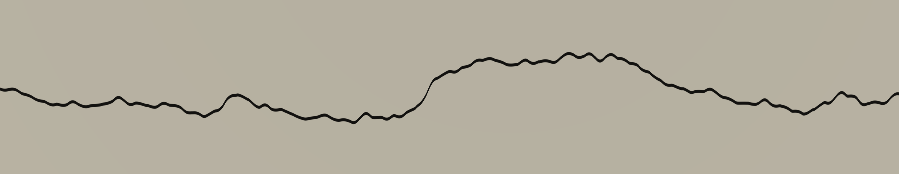## Layered multidimensional Noise

In multiple dimensions we basically do the same process, we just have to keep in mind that we have to use the appropriate vector type for the input. This is the sampling function for 2d noise:

``````float sampleLayeredNoise(float2 value){
float noise = 0;
float frequency = 1;
float factor = 1;

[unroll]
for(int i=0; i<OCTAVES; i++){
noise = noise + perlinNoise(value * frequency + i * 0.72354) * factor;
factor *= _Persistance;
frequency *= _Roughness;
}

return noise;
}
``````

And this is for 3d noise:

``````float sampleLayeredNoise(float3 value){
float noise = 0;
float frequency = 1;
float factor = 1;

[unroll]
for(int i=0; i<OCTAVES; i++){
noise = noise + perlinNoise(value * frequency + i * 0.72354) * factor;
factor *= _Persistance;
frequency *= _Roughness;
}

return noise;
}
``````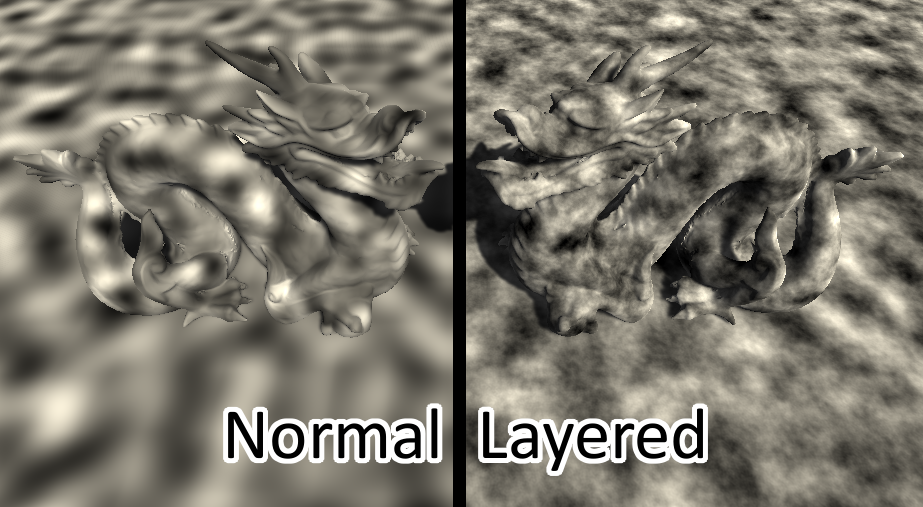## Special Use Case

Another thing noise is used for frequently is as a heightmap. For that we simply read the noise in the vertex shader instead of the fragment shader and add it to our vertex. In this part I will reference an earlier tutorial about displacement, so if you have problems with this part I recommend reading this one first.

We first change our surface definition to include a vertex function as well as generate a custom shadow pass based on that `#pragma surface surf Standard fullforwardshadows vertex:vert addshadow`. Then we fill that new vertex function. Unity doesn’t pass us a world position automatically if we write a custom vertex function, but we can calculate it ourselved my simply multiplying the object to world matrix with the local vertex position. After sampling the noise like we’re used to we add it to the vertex position in the up(Y) axis. Because we’re not dealing with colors anymore I’m also going to add a amplitude variable which will allow us to set the strength of the noise. We’ll simply multiply the noise with the amplitude before applying it.

Now that we’re displaying the noise as hight, we’ll stop writing it in the fragment shader and instead just set the albedo color to white.

If we want to see all of the detail, we have to use a high resolution mesh in this case, otherwise there wouldn’t be that many polygons for the shader to translate.

``````Properties {
_CellSize ("Cell Size", Range(0, 10)) = 2
_Roughness ("Roughness", Range(1, 8)) = 3
_Persistance ("Persistance", Range(0, 1)) = 0.4
_Amplitude("Amplitude", Range(0, 10)) = 1
}
``````
``````//global shader variables
float _Amplitude;
``````
``````void vert(inout appdata_full data){
float4 worldPos = mul(unity_ObjectToWorld, data.vertex);
float3 value = worldPos / _CellSize;
//get noise and adjust it to be ~0-1 range
float noise = sampleLayeredNoise(value) + 0.5;
data.vertex.y += noise * _Amplitude;
}
``````
``````void surf (Input i, inout SurfaceOutputStandard o) {
o.Albedo = 1;
}
``````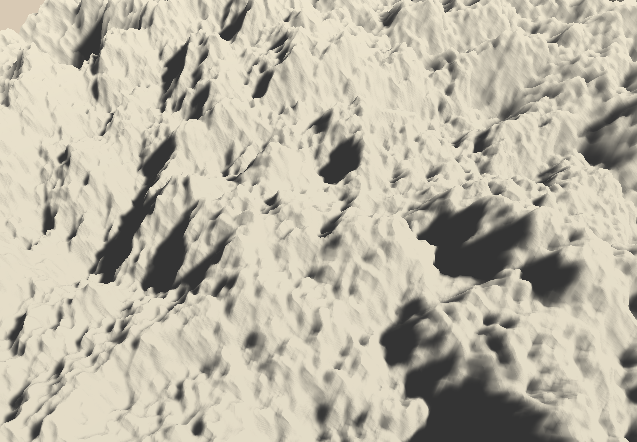The problem with this so far is that we only changed the position of the vertices, but the mesh still thinks it’s a flat surface pointing up. The shadows we see are all thrown shadows where the mesh throws a shadow on itself. We can improve that by recalculating the shadows based on the new positions. (that part is explained in the displacement tutorial)

While making this tutorial I ran into the problem that the `w` component of the vector wasn’t 1, which means that the xyz components don’t represent the actual lengths. To fix that I had to divide the xyz components by the w component before using them.

``````void vert(inout appdata_full data){
//get real base position
float3 localPos = data.vertex / data.vertex.w;

//calculate new posiiton
float3 modifiedPos = localPos;
float2 basePosValue = mul(unity_ObjectToWorld, modifiedPos).xz / _CellSize;
float basePosNoise = sampleLayeredNoise(basePosValue) + 0.5;
modifiedPos.y += basePosNoise * _Amplitude;

//calculate new position based on pos + tangent
float3 posPlusTangent = localPos + data.tangent * 0.02;
float2 tangentPosValue = mul(unity_ObjectToWorld, posPlusTangent).xz / _CellSize;
float tangentPosNoise = sampleLayeredNoise(tangentPosValue) + 0.5;
posPlusTangent.y += tangentPosNoise * _Amplitude;

//calculate new position based on pos + bitangent
float3 bitangent = cross(data.normal, data.tangent);
float3 posPlusBitangent = localPos + bitangent * 0.02;
float2 bitangentPosValue = mul(unity_ObjectToWorld, posPlusBitangent).xz / _CellSize;
float bitangentPosNoise = sampleLayeredNoise(bitangentPosValue) + 0.5;
posPlusBitangent.y += bitangentPosNoise * _Amplitude;

//get recalculated tangent and bitangent
float3 modifiedTangent = posPlusTangent - modifiedPos;
float3 modifiedBitangent = posPlusBitangent - modifiedPos;

//calculate new normal and set position + normal
float3 modifiedNormal = cross(modifiedTangent, modifiedBitangent);
data.normal = normalize(modifiedNormal);
data.vertex = float4(modifiedPos.xyz, 1);
}
``````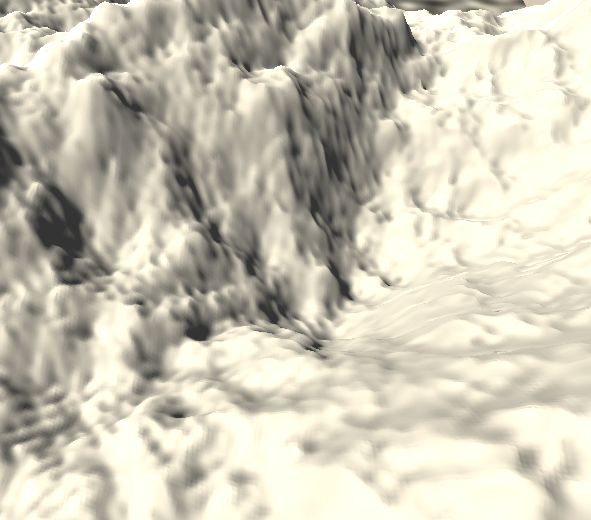Now that we did this we can also make it scroll, just to look nice. For that we’ll just add the vector multiplied by the time to the all input values.

``````//Properties
_ScrollDirection("Scroll Direction", Vector) = (0, 1)
``````
``````//global shader variables
float2 _ScrollDirection;
``````
``````//calculate base position
float2 basePosValue = mul(unity_ObjectToWorld, modifiedPos).xz / _CellSize + _ScrollDirection * _Time.y;
``````
``````//calculate tangent position
float2 tangentPosValue = mul(unity_ObjectToWorld, posPlusTangent).xz / _CellSize + _ScrollDirection * _Time.y;
``````
``````//calculate bitangent position
float2 bitangentPosValue = mul(unity_ObjectToWorld, posPlusBitangent).xz / _CellSize + _ScrollDirection * _Time.y;
``````## Source

### 1d layered noise

``````Shader "Tutorial/027_layered_noise/1d" {
Properties {
_CellSize ("Cell Size", Range(0, 2)) = 2
_Roughness ("Roughness", Range(1, 8)) = 3
_Persistance ("Persistance", Range(0, 1)) = 0.4
}
Tags{ "RenderType"="Opaque" "Queue"="Geometry"}

CGPROGRAM

#pragma target 3.0

#include "Random.cginc"

#define OCTAVES 4

float _CellSize;
float _Roughness;
float _Persistance;

struct Input {
float3 worldPos;
};

float easeIn(float interpolator){
return interpolator * interpolator * interpolator * interpolator * interpolator;
}

float easeOut(float interpolator){
return 1 - easeIn(1 - interpolator);
}

float easeInOut(float interpolator){
float easeInValue = easeIn(interpolator);
float easeOutValue = easeOut(interpolator);
return lerp(easeInValue, easeOutValue, interpolator);
}

float fraction = frac(value);
float interpolator = easeInOut(fraction);

float previousCellInclination = rand1dTo1d(floor(value)) * 2 - 1;
float previousCellLinePoint = previousCellInclination * fraction;

float nextCellInclination = rand1dTo1d(ceil(value)) * 2 - 1;
float nextCellLinePoint = nextCellInclination * (fraction - 1);

return lerp(previousCellLinePoint, nextCellLinePoint, interpolator);
}

float sampleLayeredNoise(float value){
float noise = 0;
float frequency = 1;
float factor = 1;

[unroll]
for(int i=0; i<OCTAVES; i++){
noise = noise + gradientNoise(value * frequency + i * 0.72354) * factor;
factor *= _Persistance;
frequency *= _Roughness;
}

return noise;
}

void surf (Input i, inout SurfaceOutputStandard o) {
float value = i.worldPos.x / _CellSize;
float noise = sampleLayeredNoise(value);

float dist = abs(noise - i.worldPos.y);
float pixelHeight = fwidth(i.worldPos.y);
float lineIntensity = smoothstep(2*pixelHeight, pixelHeight, dist);
o.Albedo = lerp(1, 0, lineIntensity);
}
ENDCG
}
FallBack "Standard"
}
``````

### 2d layered noise

``````Shader "Tutorial/027_layered_noise/2d" {
Properties {
_CellSize ("Cell Size", Range(0, 2)) = 2
_Roughness ("Roughness", Range(1, 8)) = 3
_Persistance ("Persistance", Range(0, 1)) = 0.4
}
Tags{ "RenderType"="Opaque" "Queue"="Geometry"}

CGPROGRAM

#pragma target 3.0

#include "Random.cginc"

#define OCTAVES 4

float _CellSize;
float _Roughness;
float _Persistance;

struct Input {
float3 worldPos;
};

float easeIn(float interpolator){
return interpolator * interpolator;
}

float easeOut(float interpolator){
return 1 - easeIn(1 - interpolator);
}

float easeInOut(float interpolator){
float easeInValue = easeIn(interpolator);
float easeOutValue = easeOut(interpolator);
return lerp(easeInValue, easeOutValue, interpolator);
}

float perlinNoise(float2 value){
//generate random directions
float2 lowerLeftDirection = rand2dTo2d(float2(floor(value.x), floor(value.y))) * 2 - 1;
float2 lowerRightDirection = rand2dTo2d(float2(ceil(value.x), floor(value.y))) * 2 - 1;
float2 upperLeftDirection = rand2dTo2d(float2(floor(value.x), ceil(value.y))) * 2 - 1;
float2 upperRightDirection = rand2dTo2d(float2(ceil(value.x), ceil(value.y))) * 2 - 1;

float2 fraction = frac(value);

//get values of cells based on fraction and cell directions
float lowerLeftFunctionValue = dot(lowerLeftDirection, fraction - float2(0, 0));
float lowerRightFunctionValue = dot(lowerRightDirection, fraction - float2(1, 0));
float upperLeftFunctionValue = dot(upperLeftDirection, fraction - float2(0, 1));
float upperRightFunctionValue = dot(upperRightDirection, fraction - float2(1, 1));

float interpolatorX = easeInOut(fraction.x);
float interpolatorY = easeInOut(fraction.y);

//interpolate between values
float lowerCells = lerp(lowerLeftFunctionValue, lowerRightFunctionValue, interpolatorX);
float upperCells = lerp(upperLeftFunctionValue, upperRightFunctionValue, interpolatorX);

float noise = lerp(lowerCells, upperCells, interpolatorY);
return noise;
}

float sampleLayeredNoise(float2 value){
float noise = 0;
float frequency = 1;
float factor = 1;

[unroll]
for(int i=0; i<OCTAVES; i++){
noise = noise + perlinNoise(value * frequency + i * 0.72354) * factor;
factor *= _Persistance;
frequency *= _Roughness;
}

return noise;
}

void surf (Input i, inout SurfaceOutputStandard o) {
float2 value = i.worldPos.xz / _CellSize;
//get noise and adjust it to be ~0-1 range
float noise = sampleLayeredNoise(value) + 0.5;

o.Albedo = noise;
}
ENDCG
}
FallBack "Standard"
}
``````

### 3d layered noise

``````Shader "Tutorial/027_layered_noise/3d" {
Properties {
_CellSize ("Cell Size", Range(0, 2)) = 2
_Roughness ("Roughness", Range(1, 8)) = 3
_Persistance ("Persistance", Range(0, 1)) = 0.4
}
Tags{ "RenderType"="Opaque" "Queue"="Geometry"}

CGPROGRAM

#pragma target 3.0

#include "Random.cginc"

#define OCTAVES 4

float _CellSize;
float _Roughness;
float _Persistance;

struct Input {
float3 worldPos;
};

float easeIn(float interpolator){
return interpolator * interpolator;
}

float easeOut(float interpolator){
return 1 - easeIn(1 - interpolator);
}

float easeInOut(float interpolator){
float easeInValue = easeIn(interpolator);
float easeOutValue = easeOut(interpolator);
return lerp(easeInValue, easeOutValue, interpolator);
}

float perlinNoise(float3 value){
float3 fraction = frac(value);

float interpolatorX = easeInOut(fraction.x);
float interpolatorY = easeInOut(fraction.y);
float interpolatorZ = easeInOut(fraction.z);

float3 cellNoiseZ;
[unroll]
for(int z=0;z<=1;z++){
float3 cellNoiseY;
[unroll]
for(int y=0;y<=1;y++){
float3 cellNoiseX;
[unroll]
for(int x=0;x<=1;x++){
float3 cell = floor(value) + float3(x, y, z);
float3 cellDirection = rand3dTo3d(cell) * 2 - 1;
float3 compareVector = fraction - float3(x, y, z);
cellNoiseX[x] = dot(cellDirection, compareVector);
}
cellNoiseY[y] = lerp(cellNoiseX, cellNoiseX, interpolatorX);
}
cellNoiseZ[z] = lerp(cellNoiseY, cellNoiseY, interpolatorY);
}
float3 noise = lerp(cellNoiseZ, cellNoiseZ, interpolatorZ);
return noise;
}

float sampleLayeredNoise(float3 value){
float noise = 0;
float frequency = 1;
float factor = 1;

[unroll]
for(int i=0; i<OCTAVES; i++){
noise = noise + perlinNoise(value * frequency + i * 0.72354) * factor;
factor *= _Persistance;
frequency *= _Roughness;
}

return noise;
}

void surf (Input i, inout SurfaceOutputStandard o) {
float3 value = i.worldPos / _CellSize;
//get noise and adjust it to be ~0-1 range
float noise = sampleLayeredNoise(value) + 0.5;

o.Albedo = noise;
}
ENDCG
}
FallBack "Standard"
}
``````

### Scrolling height noise

``````Shader "Tutorial/027_layered_noise/special_use_case" {
Properties {
_CellSize ("Cell Size", Range(0, 16)) = 2
_Roughness ("Roughness", Range(1, 8)) = 3
_Persistance ("Persistance", Range(0, 1)) = 0.4
_Amplitude("Amplitude", Range(0, 10)) = 1
_ScrollDirection("Scroll Direction", Vector) = (0, 1, 0, 0)
}
Tags{ "RenderType"="Opaque" "Queue"="Geometry"}

CGPROGRAM

#pragma target 3.0

#include "Random.cginc"

#define OCTAVES 4

float _CellSize;
float _Roughness;
float _Persistance;
float _Amplitude;

float2 _ScrollDirection;

struct Input {
float3 worldPos;
};

float easeIn(float interpolator){
return interpolator * interpolator;
}

float easeOut(float interpolator){
return 1 - easeIn(1 - interpolator);
}

float easeInOut(float interpolator){
float easeInValue = easeIn(interpolator);
float easeOutValue = easeOut(interpolator);
return lerp(easeInValue, easeOutValue, interpolator);
}

float perlinNoise(float2 value){
//generate random directions
float2 lowerLeftDirection = rand2dTo2d(float2(floor(value.x), floor(value.y))) * 2 - 1;
float2 lowerRightDirection = rand2dTo2d(float2(ceil(value.x), floor(value.y))) * 2 - 1;
float2 upperLeftDirection = rand2dTo2d(float2(floor(value.x), ceil(value.y))) * 2 - 1;
float2 upperRightDirection = rand2dTo2d(float2(ceil(value.x), ceil(value.y))) * 2 - 1;

float2 fraction = frac(value);

//get values of cells based on fraction and cell directions
float lowerLeftFunctionValue = dot(lowerLeftDirection, fraction - float2(0, 0));
float lowerRightFunctionValue = dot(lowerRightDirection, fraction - float2(1, 0));
float upperLeftFunctionValue = dot(upperLeftDirection, fraction - float2(0, 1));
float upperRightFunctionValue = dot(upperRightDirection, fraction - float2(1, 1));

float interpolatorX = easeInOut(fraction.x);
float interpolatorY = easeInOut(fraction.y);

//interpolate between values
float lowerCells = lerp(lowerLeftFunctionValue, lowerRightFunctionValue, interpolatorX);
float upperCells = lerp(upperLeftFunctionValue, upperRightFunctionValue, interpolatorX);

float noise = lerp(lowerCells, upperCells, interpolatorY);
return noise;
}

float sampleLayeredNoise(float2 value){
float noise = 0;
float frequency = 1;
float factor = 1;

[unroll]
for(int i=0; i<OCTAVES; i++){
noise = noise + perlinNoise(value * frequency + i * 0.72354) * factor;
factor *= _Persistance;
frequency *= _Roughness;
}

return noise;
}

void vert(inout appdata_full data){
//get real base position
float3 localPos = data.vertex / data.vertex.w;

//calculate new posiiton
float3 modifiedPos = localPos;
float2 basePosValue = mul(unity_ObjectToWorld, modifiedPos).xz / _CellSize + _ScrollDirection * _Time.y;
float basePosNoise = sampleLayeredNoise(basePosValue) + 0.5;
modifiedPos.y += basePosNoise * _Amplitude;

//calculate new position based on pos + tangent
float3 posPlusTangent = localPos + data.tangent * 0.02;
float2 tangentPosValue = mul(unity_ObjectToWorld, posPlusTangent).xz / _CellSize + _ScrollDirection * _Time.y;
float tangentPosNoise = sampleLayeredNoise(tangentPosValue) + 0.5;
posPlusTangent.y += tangentPosNoise * _Amplitude;

//calculate new position based on pos + bitangent
float3 bitangent = cross(data.normal, data.tangent);
float3 posPlusBitangent = localPos + bitangent * 0.02;
float2 bitangentPosValue = mul(unity_ObjectToWorld, posPlusBitangent).xz / _CellSize + _ScrollDirection * _Time.y;
float bitangentPosNoise = sampleLayeredNoise(bitangentPosValue) + 0.5;
posPlusBitangent.y += bitangentPosNoise * _Amplitude;

//get recalculated tangent and bitangent
float3 modifiedTangent = posPlusTangent - modifiedPos;
float3 modifiedBitangent = posPlusBitangent - modifiedPos;

//calculate new normal and set position + normal
float3 modifiedNormal = cross(modifiedTangent, modifiedBitangent);
data.normal = normalize(modifiedNormal);
data.vertex = float4(modifiedPos.xyz, 1);
}

void surf (Input i, inout SurfaceOutputStandard o) {
o.Albedo = 1;
}
ENDCG
}
FallBack "Standard"
}
``````

Octaves are a great way to add complexity to your noise patterns. You can also try to use the same technique to mix different kinds of noise and see what happens. In any case I hope that you learned how it works and you can do amazing stuff with it. If you feel like something is missing or confusing, just write me.

You can also find me on twitter at @totallyRonja. If you liked my tutorial and want to support me you can do that on Patreon (patreon.com/RonjaTutorials) or Ko-Fi (ko-fi.com/RonjaTutorials).